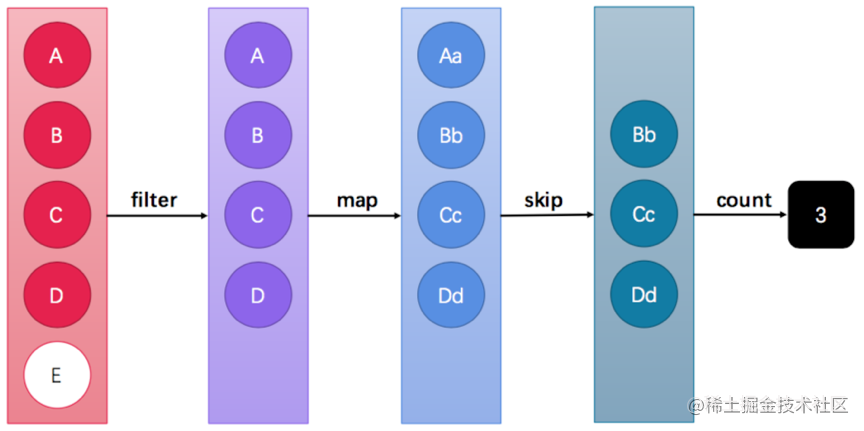# 第三章 Stream流

## 3.1 引言

``````public class Demo10ForEach {
public static void main(String[] args) {
List<String> list = new ArrayList<>();
for (String name : list) {
System.out.println(name);
}
}
}

Java 8的Lambda让我们可以更加专注于做什么（What），而不是怎么做（How），这点此前已经结合内部类进行了对比说明。现在，我们仔细体会一下上例代码，可以发现：

• for循环的语法就是“怎么做
• for循环的循环体才是“做什么

1. 将集合A根据条件一过滤为子集B
2. 然后再根据条件二过滤为子集C

1. 首先筛选所有姓张的人；
2. 然后筛选名字有三个字的人；
3. 最后进行对结果进行打印输出。
``````public class Demo11NormalFilter {
public static void main(String[] args) {
List<String> list = new ArrayList<>();

List<String> zhangList = new ArrayList<>();
for (String name : list) {
if (name.startsWith("张")) {
}
}

List<String> shortList = new ArrayList<>();
for (String name : zhangList) {
if (name.length() == 3) {
}
}

for (String name : shortList) {
System.out.println(name);
}
}
}

Stream的更优写法

``````public class Demo12StreamFilter {
public static void main(String[] args) {
List<String> list = new ArrayList<>();

list.stream()
.filter(s -> s.startsWith("张"))
.filter(s -> s.length() == 3)
.forEach(s -> System.out.println(s));
}
}

## 3.2 流式思想概述## 3.3 获取流方式

`java.util.stream.Stream<T>`是Java 8新加入的最常用的流接口。（这并不是一个函数式接口。）

• 所有的`Collection`集合都可以通过`stream`默认方法获取流；
• `Stream`接口的静态方法`of`可以获取数组对应的流。

``````import java.util.*;
import java.util.stream.Stream;
/*
获取Stream流的方式

1.Collection中 方法
Stream stream()
2.Stream接口 中静态方法
of(T...t) 向Stream中添加多个数据
*/
public class Demo13GetStream {
public static void main(String[] args) {
List<String> list = new ArrayList<>();
// ...
Stream<String> stream1 = list.stream();

Set<String> set = new HashSet<>();
// ...
Stream<String> stream2 = set.stream();
}
}

``````import java.util.stream.Stream;

public class Demo14GetStream {
public static void main(String[] args) {
String[] array = { "张无忌", "张翠山", "张三丰", "张一元" };
Stream<String> stream = Stream.of(array);
}
}

## 3.4 常用方法

• 终结方法：返回值类型不再是`Stream`接口自身类型的方法，因此不再支持类似`StringBuilder`那样的链式调用。本小节中，终结方法包括`count``forEach`方法。
• 非终结方法：返回值类型仍然是`Stream`接口自身类型的方法，因此支持链式调用。（除了终结方法外，其余方法均为非终结方法。）

### forEach : 逐一处理

``````void forEach(Consumer<? super T> action);

``````import java.util.stream.Stream;

public class Demo15StreamForEach {
public static void main(String[] args) {
Stream<String> stream =  Stream.of("大娃","二娃","三娃","四娃","五娃","六娃","七娃","爷爷","蛇精","蝎子精");
//Stream<String> stream = Stream.of("张无忌", "张三丰", "周芷若");
stream.forEach((String str)->{System.out.println(str);});
}
}

### filter：过滤

``````Stream<T> filter(Predicate<? super T> predicate);

Stream流中的`filter`方法基本使用的代码如：

``````public class Demo16StreamFilter {
public static void main(String[] args) {
Stream<String> original = Stream.of("张无忌", "张三丰", "周芷若");
Stream<String> result = original.filter((String s) -> {return s.startsWith("张");});
}
}

### count：统计个数

``````long count();

``````public class Demo17StreamCount {
public static void main(String[] args) {
Stream<String> original = Stream.of("张无忌", "张三丰", "周芷若");
Stream<String> result = original.filter(s -> s.startsWith("张"));
System.out.println(result.count()); // 2
}
}

### limit：取用前几个

`limit`方法可以对流进行截取，只取用前n个。方法签名：

``````Stream<T> limit(long maxSize):获取Stream流对象中的前n个元素,返回一个新的Stream流对象

``````import java.util.stream.Stream;

public class Demo18StreamLimit {
public static void main(String[] args) {
Stream<String> original = Stream.of("张无忌", "张三丰", "周芷若");
Stream<String> result = original.limit(2);
System.out.println(result.count()); // 2
}
}

### skip：跳过前几个

``````Stream<T> skip(long n): 跳过Stream流对象中的前n个元素,返回一个新的Stream流对象

``````import java.util.stream.Stream;

public class Demo19StreamSkip {
public static void main(String[] args) {
Stream<String> original = Stream.of("张无忌", "张三丰", "周芷若");
Stream<String> result = original.skip(2);
System.out.println(result.count()); // 1
}
}

### concat：组合

``````static <T> Stream<T> concat(Stream<? extends T> a, Stream<? extends T> b): 把参数列表中的两个Stream流对象a和b,合并成一个新的Stream流对象

``````import java.util.stream.Stream;

public class Demo20StreamConcat {
public static void main(String[] args) {
Stream<String> streamA = Stream.of("张无忌");
Stream<String> streamB = Stream.of("张翠山");
Stream<String> result = Stream.concat(streamA, streamB);
}
}

## 3.5 Stream综合案例

1. 第一个队伍只要名字为3个字的成员姓名；
2. 第一个队伍筛选之后只要前3个人；
3. 第二个队伍只要姓张的成员姓名；
4. 第二个队伍筛选之后不要前2个人；
5. 将两个队伍合并为一个队伍；
6. 打印整个队伍的姓名信息。

``````public class Demo21ArrayListNames {
public static void main(String[] args) {
List<String> one = new ArrayList<>();

List<String> two = new ArrayList<>();
// ....
}
}

``````public class Demo22ArrayListNames {
public static void main(String[] args) {
List<String> one = new ArrayList<>();
// ...

List<String> two = new ArrayList<>();
// ...

// 第一个队伍只要名字为3个字的成员姓名；
List<String> oneA = new ArrayList<>();
for (String name : one) {
if (name.length() == 3) {
}
}

// 第一个队伍筛选之后只要前3个人；
List<String> oneB = new ArrayList<>();
for (int i = 0; i < 3; i++) {
}

// 第二个队伍只要姓张的成员姓名；
List<String> twoA = new ArrayList<>();
for (String name : two) {
if (name.startsWith("张")) {
}
}

// 第二个队伍筛选之后不要前2个人；
List<String> twoB = new ArrayList<>();
for (int i = 2; i < twoA.size(); i++) {
}

// 将两个队伍合并为一个队伍；
List<String> totalNames = new ArrayList<>();

// 打印整个队伍的姓名信息。
for (String name : totalNames) {
System.out.println(name);
}
}
}

``````宋远桥

Stream方式

``````public class Demo23StreamNames {
public static void main(String[] args) {
List<String> one = new ArrayList<>();
// ...

List<String> two = new ArrayList<>();
// ...

// 第一个队伍只要名字为3个字的成员姓名；
// 第一个队伍筛选之后只要前3个人；
Stream<String> streamOne = one.stream().filter(s -> s.length() == 3).limit(3);

// 第二个队伍只要姓张的成员姓名；
// 第二个队伍筛选之后不要前2个人；
Stream<String> streamTwo = two.stream().filter(s -> s.startsWith("张")).skip(2);

// 将两个队伍合并为一个队伍；
// 根据姓名创建Person对象；
// 打印整个队伍的Person对象信息。
Stream.concat(streamOne, streamTwo).forEach(s->System.out.println(s));
}
}

``````宋远桥

count统计个数终结
forEach逐一处理终结
filter过滤函数拼接
limit取用前几个函数拼接
skip跳过前几个函数拼接
concat组合函数拼接

# 第四章 方法引用

## 4.1 概述和方法引用符

``````public class DemoPrintSimple {
private static void printString(Consumer<String> data, String str) {
data.accept(str);
}
public static void main(String[] args) {
printString(s -> System.out.println(s), "Hello World");
}
}

**应用场景 : **如果Lambda要表达的函数方案 , 已经存在于某个方法的实现中，那么则可以使用方法引用。

• Lambda表达式写法：s -> System.out.println(s); 拿到参数之后经Lambda之手，继而传递给System.out.println方法去处理。
• 方法引用写法：System.out::println 直接让System.out中的println方法来取代Lambda。

**推导与省略 : ** 如果使用Lambda，那么根据“可推导就是可省略”的原则，无需指定参数类型，也无需指定的重载形式——它们都将被自动推导。而如果使用方法引用，也是同样可以根据上下文进行推导。函数式接口是Lambda的基础，而方法引用是Lambda的简化形式。

## 4.2 方法引用简化

``````public class DemoPrintRef {
private static void printString(Consumer<String> data, String str) {
data.accept(str);
}
public static void main(String[] args) {
printString(System.out::println, "HelloWorld");
}
}

## 4.3 扩展的引用方式

### 对象名--引用成员方法

``````public class DemoMethodRef {
public static void main(String[] args) {
String str = "hello";
printUP(str::toUpperCase);
}

public static void printUP(Supplier< String> sup ){
String apply =sup.get();
System.out.println(apply);
}
}

### 类名--引用静态方法

``````public class DemoMethodRef {
public static void main(String[] args) {
printRanNum(Math::random);
}

public static void printRanNum(Supplier<Double> sup ){
Double apply =sup.get();
System.out.println(apply);
}
}

• Lambda表达式：`n -> Math.abs(n)`
• 方法引用：`Math::abs`

### 类--构造引用

``````public class Person {
private String name;
public Person(String name) {
this.name = name;
}
public String getName() {
return name;
}
}

``````public class Demo09Lambda {
public static void main(String[] args) {
String name = "tom";
Person person = createPerson(Person::new, name);
System.out.println(person);

}

public static Person createPerson(Function<String, Person> fun , String name){
Person p = fun.apply(name);
return p;

}
}

• Lambda表达式：`name -> new Person(name)`
• 方法引用：`Person::new`

### 数组--构造引用

``````public class Demo11ArrayInitRef {
public static void main(String[] args) {

int[] array = createArray(int[]::new, 3);
System.out.println(array.length);

}

public static int[] createArray(Function<Integer , int[]> fun , int n){
int[] p = fun.apply(n);
return p;

}
}

• Lambda表达式：`length -> new int[length]`
• 方法引用：`int[]::new`

• 欢迎留言，写下你感兴趣的技术话题，霈哥话优先编写哦~！